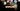# Leetcode | Solution of Valid Anagram in JavaScript

March 26th, 2020
|

In this post, we will solve the problem valid anagram from leetcode and compute the time and space complexities. Let's begin.

# Problem Statement

The question can be found at leetcode valid anagram problem.

The problem states that we need to determine if two given strings are valid anagrams of each other. This means, both of the strings will have the same characters and their count should tally.

An example would be, `anagram` and `nagaram`, both have 3as, 1n, 1g, 1r, and 1m.

# Solution

We can solve this problem using a hashmap and storing all the characters as key and their count as values. If the hashmap for both the strings is "same"(same keys and same values), we can conclude that both of the strings are anagrams.

For our solution, we'll use a slightly different approach.

• We will create an array of size 26 and fill it with zeros.
• Next, we'll loop over the characters in the first string and add their count in array. For example, if the character is a, we'll increase the count to 1 at position 0 in the array, if it's b, position will be 1 and so on.
• Then, we'll loop over the second string and decrease the character count of all the characters in the array
• After both loops are done, we should have an array with all zeros. If that's not the case, then the strings are not anagrams.

We have discussed the approach, I urge you to go ahead on leetcode and give it another try.If you are here, it means something went wrong in implementation or you are just too lazy. In any case, let's see a simple implementation of the above logic.
``````var isAnagram = function (s, t) {
const ans = new Array(26).fill(0);

for (let i = 0; i < s.length; i++) {
ans[s.charCodeAt(i) - 97]++;
}

for (let i = 0; i < t.length; i++) {
ans[t.charCodeAt(i) - 97]--;
}

for (let i = 0; i < 26; i++) {
if (ans[i] !== 0)
return false;
}

return true;
};``````

Let's look at the solution. On line 2, we create an array of size 26 and fill it with zero.

Next, we are looping over the string s, taking a character, converting it to ASCII and subtracting 97 to ensure our character's numeral representation starts at 0 so that we use it as our array index. 97 here is the ASCII value of a.

We do the same thing with the second string, the only difference is, in the first one we increase the count, in the second one we decrease it.

At last, we check if all the entries in the array are zeros. If it's positive or negative, it would mean they are not anagrams.

After submission, here's the result

``````Status: Accepted
Runtime: 64ms
Memory: 36MB``````

## Time and space complexity

### Time complexity

We are looping over the first string and second string once, so the time complexity is O(n). Technically, it would be dependent on string with the longer length, but asymptotic complexity will still be the same.

### Space complexity

We are using extra space to store character count in an array, but the size of the array is constant i.e. 26, thus, space complexity would be O(1).

# Summary

So, we solved the valid anagram problem by using an array to store the character counts. This technique is used in many problems, we'll see some more problems like these very soon.

I hope you enjoyed solving this question. This is it for this one, complete source code for this post can be found on my Github Repo. Will see you in the next one.